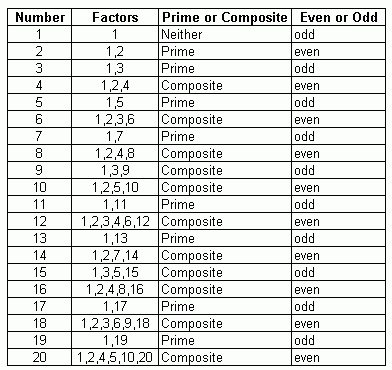Name: ___________________Date:___________________

 Email us to get an instant 20% discount on highly effective K-12 Math & English kwizNET Programs!

### Grade 5 - Mathematics1.10 Prime and Composite Facts for Numbers 1 through 20

 Prime Numbers : The numbers larger than 1 and having exactly two factors. 1 and the number itself are called prime numbers Example: 2, 3, 19, 17........ Composite Numbers : The numbers having more than two factors are called composite numbers. Example: 4, 6, 112, 150 ..........Directions: Review the following table and answer the questions. Also write atleast 10 examples of your own for prime and composite numbers.
 Q 1: Which of the following numbers is neither prime nor composite?231 Q 2: The only even prime number is ____.Answer: Q 3: _______ not considered prime numbers. 0 and 101 Q 4: How many even prime numbers are there? 21many3 Question 5: This question is available to subscribers only! Question 6: This question is available to subscribers only!